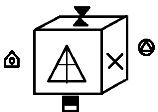# Symbol Sequence - Solved Examples

Q 1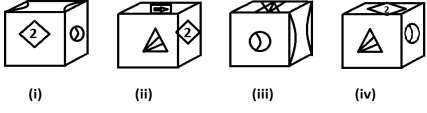Which figure is opposite to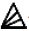?

Options :

A -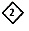B -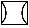C -D -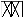Explanation

From figure (ii) and (iv), it is clear that,,, and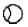will lie on adjacent face of, hencemust lie on opposite to it.

Q 2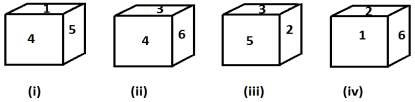Which number is opposite to number 5?

Options :

A - 3

B - 4

C - 6

D - 1

Explanation

From the figures (i) and (iii) it can be seen that 1, 2, 3, and 4 will be adjacent to 5 hence 6 must lie opposite to it.

Q 3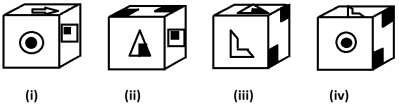Which figure is opposite to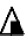?

Options :

A -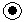B -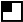C -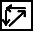D - None

Explanation

From the figures (ii) and (iii) it is clear thatwill lie opposite to...

Q 4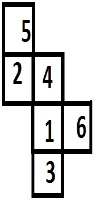Which figure will represent the question figure?

Options :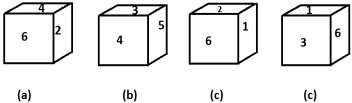Explanation

From the folded figure, it is clear that option (c) is same as the question figure.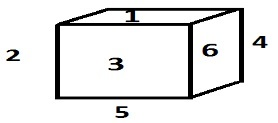Q 5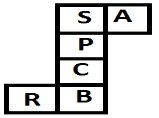Which figure is same as that of the question figure?

Options :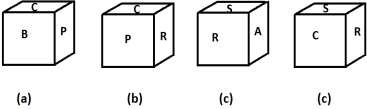Explanation

From the folded figure it is clear that option (b) is same as the question figure.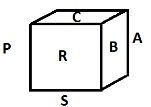Q 6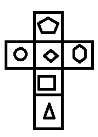Which figure can be formed by complete folding of question figure?

Options :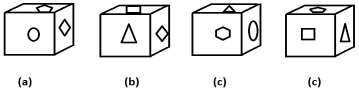Explanation

From the folded figure below it is clear that option (a) is same as the question figure.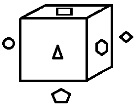Q 7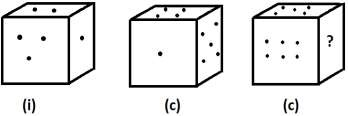Which of the following will replace question mark?

Options :

A - 2

B - 4

C - 1

D - 3

Explanation

From the figure, the unfolded figure of dice can be drawn indicating 4 will replace the question mark.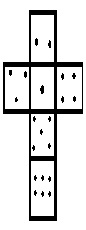Q 8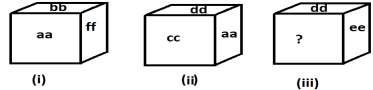Which pair of letters can be written in place of question mark?

Options :

A - cc

B - bb

C - aa

D - ff

Explanation

From the given figures it is clear that dd, cc, bb, and ff will lie on adjacent face of `aa’ therefore ‘ee’ must be opposite to it. After placing `ee’ on opposite face of `aa’, unfold figure of dice can be drawn which shows that `bb’ should placed in place of question mark.Explanation

From the unfold figure of dice it is clear that either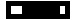or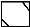will lie in place of question mark.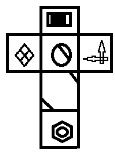Q 10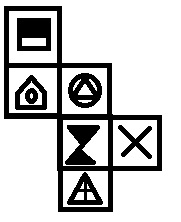The opposite figure of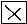is.

Options :

A -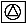B -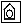C -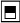D - None

From folded figure of question it clear thatis opposite to.